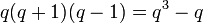# Projective general linear group of degree two

## Definition

For a field$k$, the projective general linear group of degree two$PGL(2,k)$ or$PGL_2(k)$ is defined as the quotient group of the general linear group of degree two$GL(2,k)$ by its center, which is the group of scalar matrices in it (because center of general linear group is group of scalar matrices over center).

In other words, it is the inner automorphism group of the general linear group of degree two.

The projective general linear group of degree two is the degree two special case of the projective general linear group.

For$q$ a prime power, the projective general linear group of degree two denoted$PGL(2,q)$ is defined as the projective general linear group of degree two over the field$\mathbb{F}_q$ of$q$ elements (unique up to field isomorphism).

## Arithmetic functions

### Over a finite field

Here,$q$ is the size of the finite field for which we consider the group$PGL(2,q)$.$p$ is the characteristic of the field, so$q$ is a power of$p$.

Function Value Explanation
order$\! q^3 - q = q(q - 1)(q + 1)$$|GL(2,q)| = q(q-1)^2(q+1)$, and the center has order$q - 1$, so the order of$PGL(2,q)$ is the quotient.
exponent$\! p(q^2 - 1)$ if$p = 2$,$\! p(q^2 - 1)/2$ if$p > 2$ Elements of order$p$,$q + 1$,$q - 1$, all orders divide one of these.
number of conjugacy classes$q + 1$ if$p = 2$,$q + 2$ if$p > 2$$q$ conjugacy classes of elements that come from elements not conjugate to their negative,$1$ (if$p = 2$) and$2$ (if$p > 2$) conjugacy classes coming from elements that are conjugate to their negative.

## Particular cases

### Finite fields

Note that for$q = 2$,$PGL(2,q)$ is isomorphic to all of$GL(2,q), SL(2,q), PSL(2,q)$. For$q$ a power of 2,$PGL(2,q)$ is isomorphic to$PSL(2,q)$ and$SL(2,q)$ but not to$GL(2,q)$.

For$q$ not a power of$2$,$PGL(2,q)$ admits$PSL(2,q)$ as a subgroup of index two.$SL(2,q)$ and$PGL(2,q)$ have the same order, and in fact the same composition factors, but are non-isomorphic, because$SL(2,q)$ admits$PSL(2,q)$ as a quotient rather than as a subgroup. In general, for$q \ge 5$,$PGL(2,q)$ is an almost simple group and$SL(2,q)$ is a quasisimple group.

Field size$q$ Field characteristic$p$ Exponent on$p$ giving$q$ Projective general linear group$PGL(2,q)$ Order (=$q^3 - q$) Second part of GAP ID Proof of isomorphism Comments
2 2 1 symmetric group:S3 6 1 PGL(2,2) is isomorphic to S3 not an almost simple group (one of two exceptions)
3 3 1 symmetric group:S4 24 12 PGL(2,3) is isomorphic to S4 not an almost simple group (one of two exceptions)
4 2 2 alternating group:A5 60 5 PGL(2,4) is isomorphic to A5 a simple non-abelian group (as is the case for all powers of 2 with exponent at least 2)
5 5 1 symmetric group:S5 120 34 PGL(2,5) is isomorphic to S5 an almost simple group for the simple non-abelian group alternating group:A5.
7 7 1 projective general linear group:PGL(2,7) 336 208 -- an almost simple group for the simple non-abelian group PSL(2,7) (which is isomorphic to PSL(3,2)).
8 2 3 projective special linear group:PSL(2,8) 504 156 see note above on$PSL,SL,PGL$ being isomorphic for$q$ a power of 2 a simple non-abelian group (as is the case for all powers of 2 with exponent at least 2)
9 3 2 projective general linear group:PGL(2,9) (note: this is not isomorphic to symmetric group:S6) 720 764 -- an almost simple group for the simple non-abelian group PSL(2,9), which is isomorphic to alternating group:A6. There are two other almost simple groups of the same order for$A_6$, namely symmetric group:S6 and Mathieu group:M10. These are all subgroups of the automorphism group of alternating group:A6, which is also the same as$P\Gamma L(2,9)$, which has order 1440.
11 11 1 projective general linear group:PGL(2,11) 1320 133 -- an almost simple group for projective special linear group:PSL(2,11).

## Linear representation theory

### Over a finite field

Below is a summary of the linear representation theory of$PGL(2,q)$:

Item Value
degrees of irreducible representations over a splitting field Case$q$ odd: 1 (2 times),$q - 1$ ($(q - 1)/2$ times),$q$ (2 times),$q + 1$ ($(q - 3)/2$ times)
Case$q$ even: 1 (1 time),$q - 1$ ($q/2$ times),$q$ (1 time),$q + 1$ ($(q - 2)/2$ times)
number of irreducible representations Case$q$ odd:$q + 2$, case$q$ even:$q + 1$
See number of irreducible representations equals number of conjugacy classes, element structure of projective general linear group of degree two over a finite field#Conjugacy class structure
quasirandom degree (minimum degree of nontrivial ireducible representation) 1
maximum degree of irreducible representation$q + 1$
lcm of degrees of irreducible representations Case$q$ odd:$q(q + 1)(q - 1)/2 = (q^3 - q)/2$; Case$q$ even:$q(q+1)(q-1) = q^3 - q$
sum of squares of degrees of irreducible representations$q(q + 1)(q - 1) = q^3 - q$, equal to the group order; see sum of squares of degrees of irreducible representations equals group order